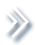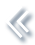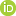####ArchivesReview Articles

## Some results of classification problem by Bayesian method and application in credit operation

Tai Vovan

College of Natural Science, Can Tho University, Can Tho, Vietnamvvtai@ctu.edu.vn

Pages 150-157 | Received 30 Nov. 2017, Accepted 22 Sep. 2018, Published online: 03 Oct. 2018,
• Abstract
• References
• Citations

ABSTRACT

This study proposes some results in classifying by Bayesian method. There are upper and lower bounds of the Bayes error as well as its determination in case of one dimension and multi-dimensions. Based on the proposals for estimating of probability density functions, calculating the Bayes error and determining the prior probability, we establish an algorithm to evaluate ability of customers to pay debts at banks. This algorithm has been performed by the Matlab procedure that can be applied well with real data. The proposed algorithm is tested by the real application at a bank in Viet Nam that obtains the best results in comparing with the existing approaches.

## References

1. Altman, D. G. (1991). Statistics in medical journals: Development in 1980s. Statistical in Medicine10, 1897–1913. doi: 10.1002/sim.4780101206 [Google Scholar]
2. Christopher, M. B. (2006). Pattern recognition and machine learning. New York, NY: Springer. [Google Scholar]
3. Cristianini, N., & Shawe, T. J. (2000). An introduction to support vector machines and other kernel-based learning method. London: Cambridge University. [Google Scholar]
4. Fisher, R. A. (1936). The statistical utilization of multiple measurements. Annals of Eugenic7, 376–386. [Google Scholar]
5. Ghosh, A. K. (2006). Classification using kernel density estimates. Technometrics48, 120–132. doi: 10.1198/004017005000000391 [Taylor & Francis Online], [Google Scholar]
6. Hastie, T., & Tibshirani, R. (1996). Discriminant adaptive nearest neighbor classification. IEEE Transactions on Pattern Analysis and Machine Intelligence18(6), 607–616. doi: 10.1109/34.506411 [Google Scholar]
7. Inman, H. F., & Bradley, E. L. (1989). The overlapping coefficient as a measure of agreement between probability distribution sand point estimation of the overlap of two normal densities. Communication in Statistics Theory and Methods18, 3851–3874. doi: 10.1080/03610928908830127 [Taylor & Francis Online], [Google Scholar]
8. James, J. (2001). Interaction effects in logistic regression. London: Sage. [Google Scholar]
9. Jan, Y. K., Cheng, C. W, & Shih, Y. H. (2010). Application of logistic regression analysis of home mortgage loan prepayment and default risk. ICIC Express Letters2, 325–331. [Google Scholar]
10. Marta, E. (2001). Application of Fisher's method to materials that only release water at high temperatures. Portugaliae Etecfochlmlca Acta15, 301–311. [Google Scholar]
11. Matusita, K. (1967). On the notion of affinity of several distributions and some of its applications. Annals of the Institute of Statistical Mathematics19(1), 181–192. doi: 10.1007/BF02911675 [Google Scholar]
12. McLachlan, G. J., & Basford, K. E. (1998). Mixture models: Inference and applications to clustering. New York, NY: Marcel Dekker. [Google Scholar]
13. Miller, G., Inkret, W. C., Little, T. T., Martz, H. F., & Schillaci, M. E. (2001). Bayesian prior probability distributions for internal dosimetry. Radiation Protection Dosimetry94, 347–352. doi: 10.1093/oxfordjournals.rpd.a006509 [Google Scholar]
14. Nguyentrang, T., & Vovan, T. (2017). Fuzzy clustering of probability density functions. Journal of Applied Statistics44(4), 583–601. doi: 10.1080/02664763.2016.1177502 [Taylor & Francis Online], [Google Scholar]
15. Nguyen-Trang, T., & Vo-Van, T. (2017). A new approach for determining the prior probabilities in the classification problem by Bayesian method. Advances in Data Analysis and Classification11, 629–643. doi: 10.1007/s11634-016-0253-y [Google Scholar]
16. Pham-Gia, T., Nhat, N. D., & Phong, N. V. (2015). Statistical classification using the maximum function. Open Journal of Applied Statistics5(7), 665–679. doi: 10.4236/ojs.2015.57068 [Google Scholar]
17. Pham–Gia, T., Turkkan, N., & Tai, V. V. (2008). Statistical discrimination analysis using the maximum function. Communications in Statistics Simulation and Computation37, 320–336. doi: 10.1080/03610910701790475 [Taylor & Francis Online], [Google Scholar]
18. Tai, V. V. (2017).- distance and classification problem by Bayesian method. Journal of Applied Statistics4(3), 385–401. [Google Scholar]
19. Tai, V. V., & Pham-Gia, T. (2010). Clustering probability distributions. Journal of Applied Statistics37(11), 1891–1910. doi: 10.1080/02664760903186049 [Taylor & Francis Online][Web of Science ®], [Google Scholar]
20. Tai, V. V., Thao, N. T., & Ha, C. N. (2016). The prior probability in classifying two populations by Bayesian method. Applied Mathematics Engineering and Reliability6, 35–40. doi: 10.1201/b21348-7 [Google Scholar]
21. Toussaint, G. T. (1972). Some inequalities between distance measures for feature. IEEE Transactions on ComputersC-21, 409–410. doi: 10.1109/TC.1972.5008991 [Google Scholar]
22. Webb, A. (2002). Statistical pattern recognition. London: John Wiley & Sons. [Google Scholar]